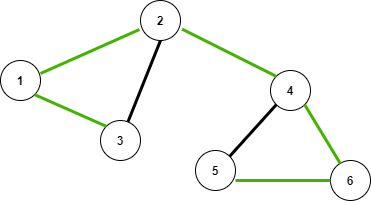# Difference between Tree edge and Back edge in graph

• Difficulty Level : Medium
• Last Updated : 13 Jan, 2021

Tree Edge: It is an edge that is present in the tree obtained after performing DFS on the graph. All the Green edges are tree edges as shown in the below image.

Back Edge: It is an edge (u, v) such that v is an ancestor of node u but not part of the DFS Traversal of the tree. Edge from 5 to 4 is a back edge. The presence of a back edge indicates a cycle in a directed graph.

Consider an undirected graph is given below, the DFS of the below graph is 3 1 2 4 6 5. In the below diagram, if the DFS is applied to this graph, a tree is obtained which is connected using green edges.Tabular between the back Edge and tree Edge:

My Personal Notes arrow_drop_up